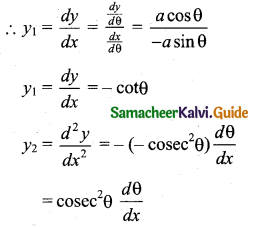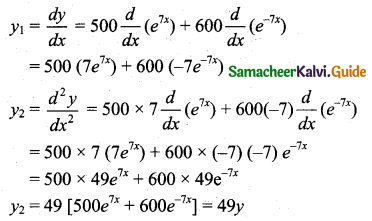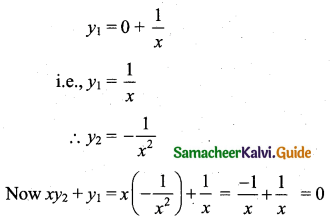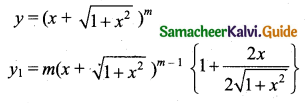Students can download 11th Business Maths Chapter 5 Differential Calculus Ex 5.9 Questions and Answers, Notes, Samcheer Kalvi 11th Business Maths Guide Pdf helps you to revise the complete Tamilnadu State Board New Syllabus, helps students complete homework assignments and to score high marks in board exams.

## Tamilnadu Samacheer Kalvi 11th Business Maths Solutions Chapter 5 Differential Calculus Ex 5.9

### Samacheer Kalvi 11th Business Maths Differential Calculus Ex 5.9 Text Book Back Questions and Answers

Question 1.
Find y2 for the following functions:
(i) y = e3x+2
(ii) y = log x + ax
(iii) x = a cosθ, y = a sinθ
Solution:
(i) y = e3x+2(ii) y = log x + ax(iii) x = a cosθ, y = a sinθ
$$\frac{d x}{d \theta}$$ = a(-sinθ) = -a sinθ …….. (i)
$$\frac{d y}{d \theta}$$ = a(cosθ)Question 2.
If y = 500e7x + 600e-7x, then show that y2 – 49y = 0.
Solution:
y = 500e7x + 600e-7x(or) y2 – 49y = 0

Question 3.
If y = 2 + log x, then show that xy2 + y1 = 0.
Solution:
y = 2 + log xQuestion 4.
If = a cos mx + b sin mx, then show that y2 + m2y = 0.
Solution:
y = a cos mx + b sin mx
y1 = a $$\frac{d}{d x}$$ (cos mx) + b $$\frac{d}{d x}$$ (sin mx)
[∵ $$\frac{d}{d x}$$ (sin mx) = cos mx $$\frac{d}{d x}$$ (mx) = (cos mx) . m]
= a(-sin mx) . m + b(cos mx) . m
= -am sin mx + bm cos mx
y2 = -am(cos mx) . m + bm(-sin mx) . m
= -am2 cos mx – bm2 sin mx
= -m2 [a cos mx + b sin mx]
= -m2y
∴ y2 + m2y = 0

Question 5.
If y = $$\left(x+\sqrt{1+x^{2}}\right)^{m}$$, then show that (1 + x2) y2 + xy1 – m2y = 0
Solution:$$y_{1}=\frac{m y}{\sqrt{1+x^{2}}}$$
Squaring both sides we get,
$$y_{1}^{2}=\frac{m^{2} y^{2}}{\left(1+x^{2}\right)}$$
(1 + x2) ($$y_{1}^{2}$$) = m2y2
Differentiating with respect to x, we get
(1 + x2) . 2(y1) (y2) + (y1)2 (2x) = 2m2yy1
Dividing both sides by 2y1 we get,
(1 + x2) y2 + xy1 = m2y
⇒ (1 + x2) y2 + xy1 – m2y = 0Question 6.
If y = sin(log x), then show that x2y2 + xy1 + y = 0.
Solution:
y = sin(log x)
y1 = cos(log x) $$\frac{d}{d x}$$ (log x)
y1 = cos(log x) . $$\frac{1}{x}$$
∴ xy1 = cos(log x)
Differentiating both sides with respect to x, we get
xy2 + y1(1) = -sin(log x) . $$\frac{1}{x}$$
⇒ x[xy2 + y1] = -sin(log x)
⇒ x2y2 + xy1 = -y
⇒ x2y2 + xy1 + y = 0